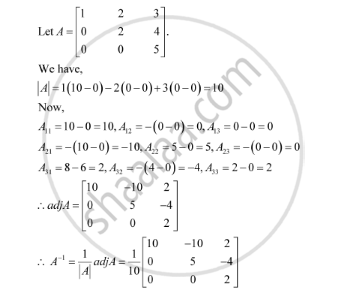Share

# Find the Inverse of Each of the Matrices (If It Exists). [(1,2,3),(0,2,4),(0,0,5)] - CBSE (Science) Class 12 - Mathematics

#### Question

Find the inverse of each of the matrices (if it exists).

[(1,2,3),(0,2,4),(0,0,5)]

#### SolutionIs there an error in this question or solution?

#### Video TutorialsVIEW ALL 

Solution Find the Inverse of Each of the Matrices (If It Exists). [(1,2,3),(0,2,4),(0,0,5)] Concept: Adjoint and Inverse of a Matrix.
S